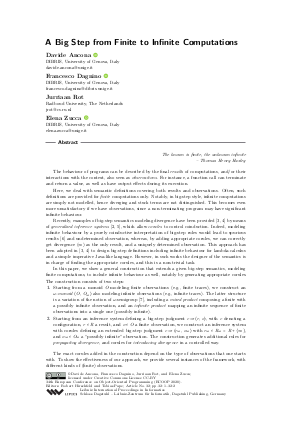Document# A Big Step from Finite to Infinite Computations (SCICO Journal-first)

### Authors Davide Ancona, Francesco Dagnino, Jurriaan Rot, Elena Zucca## File

LIPIcs.ECOOP.2020.32.pdf
• Filesize: 242 kB
• 2 pages

## Cite As

Davide Ancona, Francesco Dagnino, Jurriaan Rot, and Elena Zucca. A Big Step from Finite to Infinite Computations (SCICO Journal-first). In 34th European Conference on Object-Oriented Programming (ECOOP 2020). Leibniz International Proceedings in Informatics (LIPIcs), Volume 166, pp. 32:1-32:2, Schloss Dagstuhl - Leibniz-Zentrum für Informatik (2020)
https://doi.org/10.4230/LIPIcs.ECOOP.2020.32

## Abstract

The known is finite, the unknown infinite - Thomas Henry Huxley The behaviour of programs can be described by the final results of computations, and/or their interactions with the context, also seen as observations. For instance, a function call can terminate and return a value, as well as have output effects during its execution. Here, we deal with semantic definitions covering both results and observations. Often, such definitions are provided for finite computations only. Notably, in big-step style, infinite computations are simply not modelled, hence diverging and stuck terms are not distinguished. This becomes even more unsatisfactory if we have observations, since a non-terminating program may have significant infinite behaviour. Recently, examples of big-step semantics modeling divergence have been provided [Davide Ancona et al., 2017; Davide Ancona et al., 2018] by means of generalized inference systems [Davide Ancona et al., 2017; Francesco Dagnino, 2019], which allow corules to control coinduction. Indeed, modeling infinite behaviour by a purely coinductive interpretation of big-step rules would lead to spurious results [Xavier Leroy and Hervé Grall, 2009] and undetermined observation, whereas, by adding appropriate corules, we can correctly get divergence (∞) as the only result, and a uniquely determined observation. This approach has been adopted in [Davide Ancona et al., 2017; Davide Ancona et al., 2018] to design big-step definitions including infinite behaviour for lambda-calculus and a simple imperative Java-like language. However, in such works the designer of the semantics is in charge of finding the appropriate corules, and this is a non-trivial task. In this paper, we show a general construction that extends a given big-step semantics, modeling finite computations, to include infinite behaviour as well, notably by generating appropriate corules. The construction consists of two steps: 1) Starting from a monoid O modeling finite observations (e.g., finite traces), we construct an ω-monoid ⟨O, O_∞⟩ also modeling infinite observations (e.g., infinite traces). The latter structure is a variation of the notion of ω-semigroup [Dominique Perrin and Jean-Eric Pin, 2004], including a mixed product composing a finite with a possibly infinite observation, and an infinite product mapping an infinite sequence of finite observations into a single one (possibly infinite). 2) Starting from an inference system defining a big-step judgment c⇒⟨r, o⟩, with c denoting a configuration, r ∈ R a result, and o ∈ O a finite observation, we construct an inference system with corules defining an extended big-step judgment c⇒c ⇒ ⟨r_∞, o_∞⟩ with r_∞ ∈ R_∞ = R+{∞}, and o_∞ ∈ O_∞ a "possibly infinite" observation. The construction generates additional rules for propagating divergence, and corules for introducing divergence in a controlled way. The exact corules added in the construction depend on the type of observations that one starts with. To show the effectiveness of our approach, we provide several instances of the framework, with different kinds of (finite) observations. Finally, we prove a correctness result for the construction. To this end, we assume the original big-step semantics to be equivalent to (finite sequences of steps in) a reference small-step semantics, and we show that, by applying the construction, we obtain an extended big-step semantics which is still equivalent to the small-step semantics, where we consider possibly infinite sequences of steps.} As hypotheses, rather than {just} equivalence in the finite case {(which would be not enough)}, we assume a set of equivalence conditions between individual big-step rules and the small-step relation. This proof of equivalence holds for deterministic semantics; issues arising in the non-deterministic case and a possible solution are sketched in the conclusion of the full paper.

## Subject Classification

##### ACM Subject Classification
• Theory of computation → Operational semantics
• Software and its engineering → Recursion
• Software and its engineering → Semantics
##### Keywords
• Operational semantics
• coinduction
• infinite behaviour

## Metrics

• Access Statistics
• Total Accesses (updated on a weekly basis)
0

## References

1. Davide Ancona, Francesco Dagnino, Jurriaan Rot, and Elena Zucca. A big step from finite to infinite computations. Science of Computer Programming, page 102492, 2020. URL: https://doi.org/10.1016/j.scico.2020.102492.
2. Davide Ancona, Francesco Dagnino, and Elena Zucca. Generalizing inference systems by coaxioms. In Hongseok Yang, editor, ESOP 2017 - European Symposium on Programming, volume 10201 of Lecture Notes in Computer Science, pages 29-55. Springer, 2017. URL: https://doi.org/10.1007/978-3-662-54434-1_2.
3. Davide Ancona, Francesco Dagnino, and Elena Zucca. Reasoning on divergent computations with coaxioms. PACMPL, 1(OOPSLA):81:1-81:26, 2017. URL: https://doi.org/10.1145/3133905.
4. Davide Ancona, Francesco Dagnino, and Elena Zucca. Modeling infinite behaviour by corules. In Todd D. Millstein, editor, ECOOP'18 - Object-Oriented Programming, volume 109 of LIPIcs, pages 21:1-21:31. Schloss Dagstuhl - Leibniz-Zentrum für Informatik, 2018. URL: https://doi.org/10.4230/LIPIcs.ECOOP.2018.21.
5. Francesco Dagnino. Coaxioms: flexible coinductive definitions by inference systems. Logical Methods in Computer Science, 15(1), 2019. URL: https://doi.org/10.23638/LMCS-15(1:26)2019.
6. Xavier Leroy and Hervé Grall. Coinductive big-step operational semantics. Information and Computation, 207(2):284-304, 2009. URL: https://doi.org/10.1016/j.ic.2007.12.004.
7. Dominique Perrin and Jean-Eric Pin. Infinite words - automata, semigroups, logic and games, volume 141 of Pure and applied mathematics series. Elsevier Morgan Kaufmann, 2004.X

Feedback for Dagstuhl Publishing